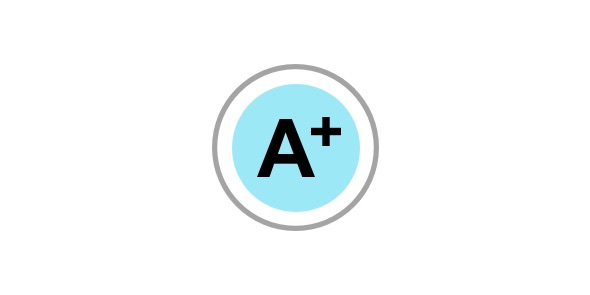# Mathematics Quiz For Grade 9

25 Questions | Total Attempts: 72Settings• 1.
A is 40% of B. what percentage is B of A?
• A.

60%

• B.

140%

• C.

250%

• D.

160%

• 2.
X+5=10. then x equals
• A.

5

• B.

10

• C.

15

• D.

-5

• 3.
X2     + 4x  +3=0     then x equals
• A.

2 or 3

• B.

-1 or -3

• C.

1 or 3

• D.

5 or -1

• 4.
3, 4,7,11, ..........   next two terms are:
• A.

16,23

• B.

18,29

• C.

17,24

• D.

19,27

• 5.
Y2+ 4=20. then y equals
• A.

4 only

• B.

-4 only

• C.

4 or -4

• D.

16

• 6.
The price of an item increases by 20% and then decreases by 20%. so the price has
• A.

Increased by 4%

• B.

Decreased by 4%

• C.

Increased by 0%

• D.

Increased by 40%

• 7.
The ratio of girls: boys in a school is 5: 7. if  there are 525 girls, how many boys are there?
• A.

375

• B.

625

• C.

735

• D.

325

• 8.
David and John share \$ 455 in the ratio 2:3 respectively. how much money does john get?
• A.

\$182

• B.

\$273

• C.

\$91

• D.

\$175

• 9.
X-12y =0. then    y/x equals
• A.

1/6

• B.

12

• C.

1/12

• D.

None

• 10.
Change 3/20 into a decimal
• A.

0.3

• B.

0.5

• C.

0.15

• D.

1.5

• 11.
Write 68 /0.00001 as an ordinary number.
• A.

6800000

• B.

680000

• C.

68000

• D.

0.00068

• 12.
4 X 4 - 2 X (-3) + 6
• A.

17

• B.

28

• C.

-36

• D.

None

• 13.
When you reduce \$ 80 by 11%, you will get:
• A.

\$8.80

• B.

\$71.20

• C.

\$69

• D.

None

• 14.
Write 0.67543 correct to 2 significant figures.
• A.

0.68

• B.

0.7

• C.

0.67

• D.

0.70

• 15.
Simplify  5ab-7ba +4 ab
• A.

2ab

• B.

-2ab

• C.

16ab

• D.

None

• 16.
Solve 4(x+2) =24
• A.

X=11/2

• B.

X=4

• C.

X=-4

• D.

X=16

• 17.
The sum of the three consecutive even numbers is 222. find the numbers.
• A.

73,74,75

• B.

74,74,74

• C.

72,74,76

• D.

None

• 18.
The gradient of the line joining (-4,-1) and (4,2)  is
• A.

-3/8

• B.

3/8

• C.

0

• D.

8/3

• 19.
Gradient of the line y = 4 -2x  is
• A.

4

• B.

2

• C.

-2

• D.

None

• 20.
Gradient of the line x+3y=9 is
• A.

3

• B.

1/3

• C.

-1/3

• D.

None

• 21.
If 2x-3y +4x-7y=0, then the value of y/x is
• A.

3/5

• B.

5/3

• C.

5/11

• D.

-3/5

• 22.
If 2x +5y=18  and x=2, what is the value of 4x+5y
• A.

20

• B.

19

• C.

22

• D.

21

• 23.
Factorise x2 +x-30
• A.

(x+5)(x-6)

• B.

(x-5)(x+6)

• C.

(x-6)(x-5)

• D.

None

• 24.
Change 36 km/h to metres per second
• A.

20

• B.

10

• C.

36

• D.

None

• 25.
A is 40% of B. what percentage is B of A?
• A.

60%

• B.

140%

• C.

250%

• D.

160%

Related TopicsBack to top